@ltlovezh 2020-04-06T03:33:06.000000Z 字数 15922 阅读 4849

# OpenGL坐标变换

OpenGL

## 基础概述

OpenGL的坐标变换流程如下所示：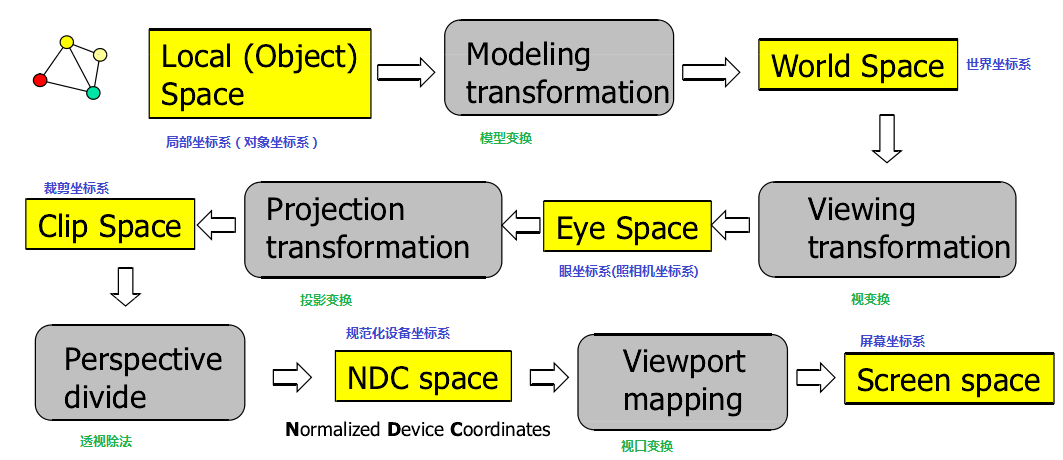1. 首先，输入顶点一般是以本地坐标表示的3D模型。本地坐标是为了研究孤立的3D模型，坐标原点一般都是模型的中心。每个3D模型都有自己的本地坐标系（Local Coordinate），互相之间没有关联。
2. 当我们需要同时渲染多个3D物体时，需要把不同的3D模型，变换到一个统一的坐标系，这就是世界坐标系（World Coordinate）。把物体从本地坐标系变换到世界坐标系，是通过一个Model矩阵完成的。模型矩阵可以实现多种变换：平移(translation)、缩放(scale)、旋转(rotation)、镜像(reflection)、错切(shear)等。例如：通过平移操作，我们可以在世界坐标系的不同位置绘制同一个3D模型；
3. 世界坐标系中的多个物体共同构成了一个3D场景。从不同的角度观察这个3D场景，我们可以看到不同的屏幕投影。OpenGL提出了摄像头坐标系的概念，即从摄像头位置来观察整个3D场景。把物体从世界坐标系变换到摄像头坐标系，是通过一个View矩阵完成的。视图矩阵定义了摄像头的位置、方向向量和上向量等构成摄像头坐标系的基础信息。View矩阵左乘世界坐标系中顶点A的坐标，就把顶点A变换到了摄像头坐标系。同一个3D物体，在世界坐标系中，拥有一个世界坐标；在摄像头坐标系中，拥有一个摄像头坐标，View变换就是负责把物体的坐标从世界坐标系变换到摄像头坐标系。
4. 因为我们是从一个2D屏幕观察3D场景，而屏幕本身不是无限大的。所以当从摄像头的角度观察3D场景时，可能无法看到整个场景，这时候就需要把看不到的场景裁减掉。投影变换就是负责裁剪工作，投影矩阵指定了一个视见体(View Frustum)，在视见体内部的物体会出现在投影平面上，而在视见体之外的物体会被裁减掉。投影包括很多类型，OpenGL中主要考虑透视投影(Perspective Projection)和正交投影(Orthographic Projection)，两者的区别在后面会详细介绍。除此之外，通过Projection矩阵，可以把物体从摄像头坐标系变换到裁剪坐标系。在裁剪坐标下，X、Y、Z各个坐标轴上会指定一个可见范围，超过可见范围的顶点(vertex)都会被裁剪掉。
5. 每个裁剪坐标系指定的可见范围可能是不同的，为了得到一个统一的坐标系，需要对裁剪坐标进行透视除法（Perspective Division），得到NDC坐标(Normalized Device Coordinates - 标准化设备坐标系)。透视除法就是将裁剪坐标除以齐次分量W，得到NDC坐标：

6. NDC坐标系的范围是[-1,1]，但是我们的屏幕尺寸是千变万化的，那么OpenGL是如何把NDC坐标映射到屏幕坐标的那？视口变换(Viewport Transform)就是负责这块工作的。在OpenGL中，我们只需要通过glViewport指定绘制区域的坐标和宽高，系统会帮我们自动完成视口变换。经过视口变换，我们就得到了2D屏幕上的屏幕坐标。需要注意的是：屏幕坐标与屏幕的像素位置是不一样的，屏幕坐标是屏幕上任意一个顶点的精确位置，可以是任意小数。但是像素位置只能是整数（具体的某个像素）。这里的视口变换是从NDC坐标变换到屏幕坐标，还没有生成最终的像素位置。从屏幕坐标映射到对应的像素位置，是后面光栅化完成的。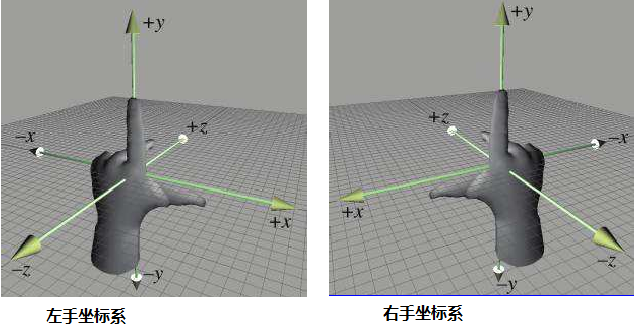## 模型变换

### 平移变换

glm::mat4 model; // 定义单位矩阵model = glm::translate(model, glm::vec3(1.0f, 1.0f, 1.0f));

### 缩放变换

1. 把顶点P移动到坐标原点
2. 以坐标原点为中心，缩放指定倍数
3. 把顶点P移动回原来的位置

glm::mat4 model; // 定义单位矩阵model = glm::scale(model, glm::vec3(2.0f, 0.5f, 1.0f);

### 旋转变换

glm::mat4 model; // 定义单位矩阵model = glm::rotate(model, glm::radians(-45.0f), glm::vec3(0.4f, 0.6f, 0.8f));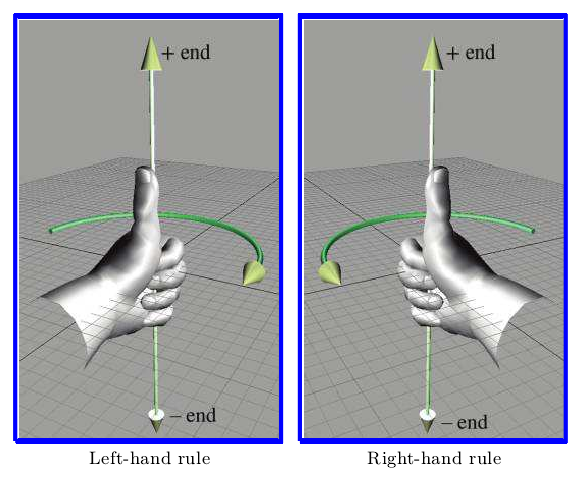### 模型变换的顺序问题

glm::mat4 model; // 定义单位矩阵model = glm::translate(model, glm::vec3(1.0f, 1.0f, 1.0f));model = glm::rotate(model, glm::radians(-45.0f), glm::vec3(0.4f, 0.6f, 0.8f));model = glm::scale(model, glm::vec3(2.0f, 0.5f, 1.0f);

## 视图变换

1. 指定坐标系的维度：2D、3D、4D等。
2. 定义坐标空间的轴向量，例如：X轴、Y轴、Z轴，这些向量称为基向量，基向量一般都是正交的。坐标系中的所有顶点都是通过基向量表示的。
3. 坐标系的原点O，原点是坐标系中所有其他点的参考点。

顶点V在A坐标系的坐标 = B坐标系的基向量和原点在A坐标系下的坐标表示构成的变换矩阵 * 顶点V在B坐标系的坐标；顶点V在B坐标系的坐标 = A坐标系的基向量和原点在B坐标系下的坐标表示构成的变换矩阵 * 顶点V在A坐标系的坐标

$[B]_A$矩阵的计算方式也类似，此处不再赘述。

1. 摄像头在世界坐标系中的位置（摄像头坐标系的原点）
2. 摄像头的观察方向（摄像头坐标系的Z基向量）
3. 一个指向摄像头右侧的向量（摄像头坐标系的X基向量）
4. 一个指向摄像头上方的向量（摄像头坐标系的Y基向量）。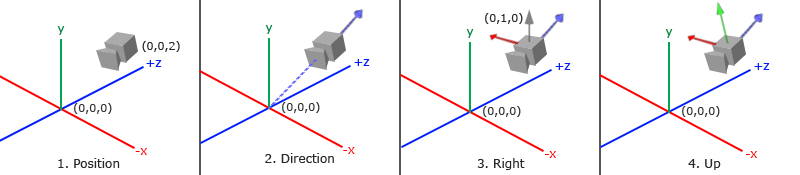1. 摄像机位置向量($\vec{eye}$)
2. 摄像机指向的目标位置向量($\vec{target}$)
3. 指向摄像头上方的向量（$\vec{up}$

1. 首先计算摄像头的方向向量$\vec{forwrad}$（方向向量是摄像头坐标系的Z轴正方向，和实际的观察方向是相反的）。

2. 根据上向量$\vec{up}$和单位方向向量$\vec{forwrad_{norm}}$确定摄像头的右向量$\vec{side}$

3. 根据单位右向量$\vec{side_{norm}}$和单位方向向量$\vec{forwrad_{norm}}$确定单位上向量$\vec{up_{norm}}$

1. $\vec{side_{norm}}$ = $\begin{bmatrix} 1 \\ 0 \\ 0 \\ \end{bmatrix}$
2. $\vec{up_{norm}}$ = $\begin{bmatrix} 0 \\ 1 \\ 0 \\ \end{bmatrix}$
3. $\vec{forward_{norm}}$ = $\begin{bmatrix} 0 \\ 0 \\ 1 \\ \end{bmatrix}$
4. $\vec{eye}$ = $\begin{bmatrix} 0 \\ 0 \\ 3 \\ \end{bmatrix}$

glm::mat4 view;view = glm::lookAt(glm::vec3(0.0f, 0.0f, 3.0f),glm::vec3(0.0f, 0.0f, 0.0f), glm::vec3(0.0f, 1.0f, 0.0f));

## 投影变换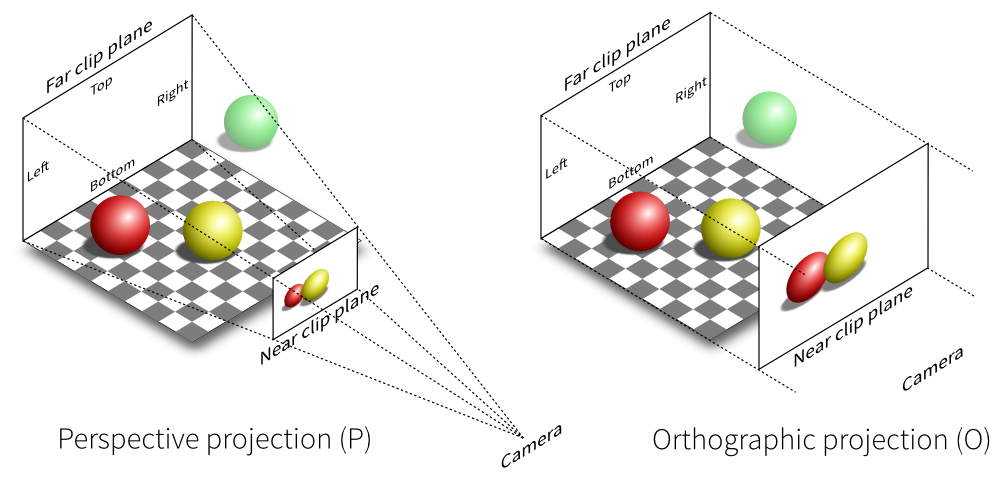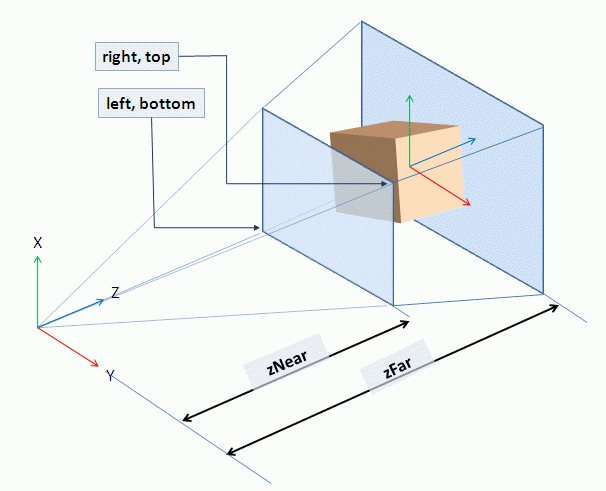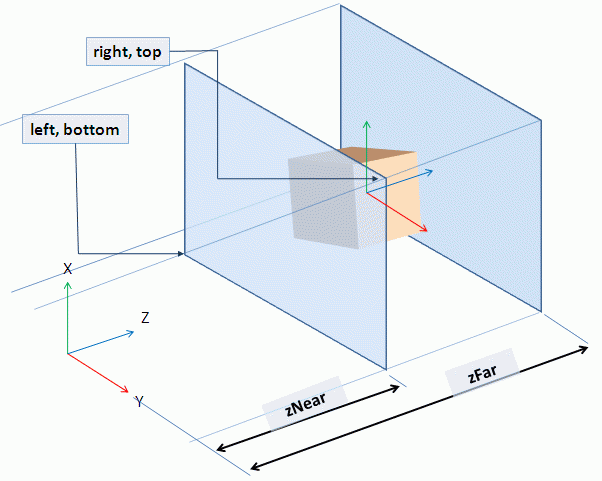### 透视投影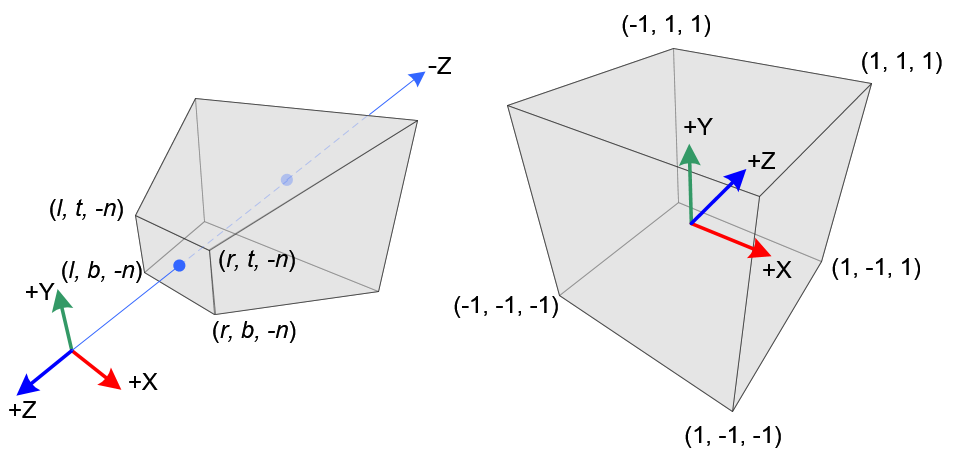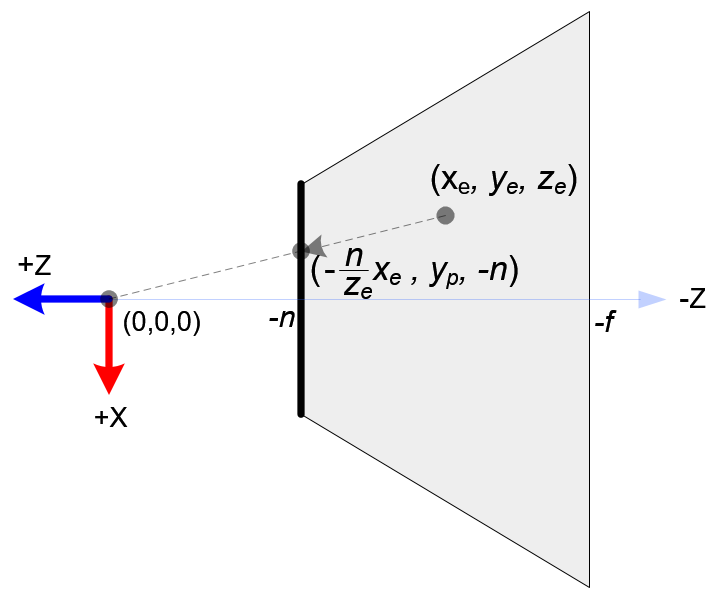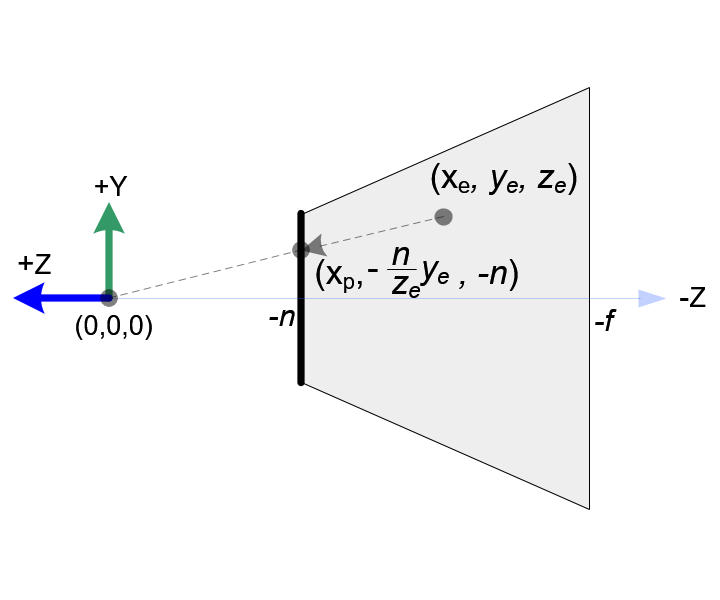$x^p$满足[left,right]映射到[-1,1]，如下所示：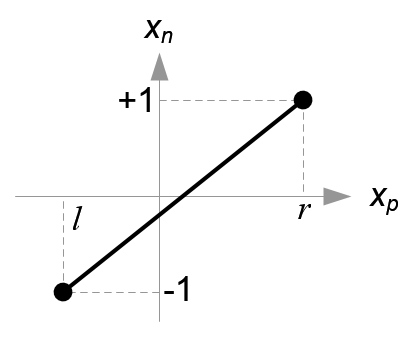OK，继续看下$y^{p}$的映射关系：满足[bottom,top]映射到[-1,1]，如下所示：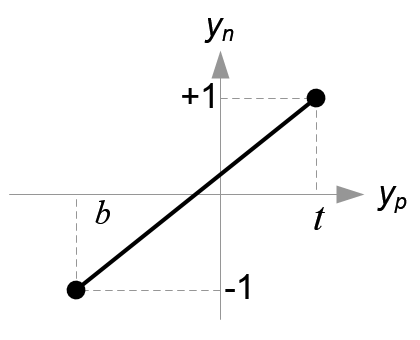glm::mat4 proj = glm::perspective(glm::radians(45.0f), width/height, 0.1f, 100.0f);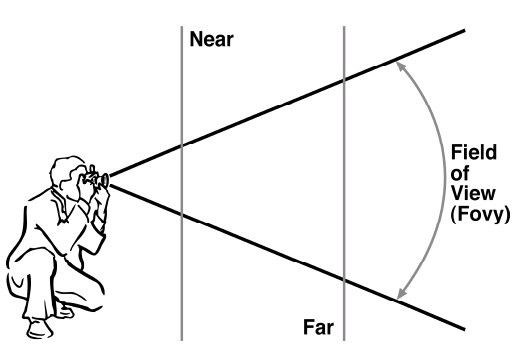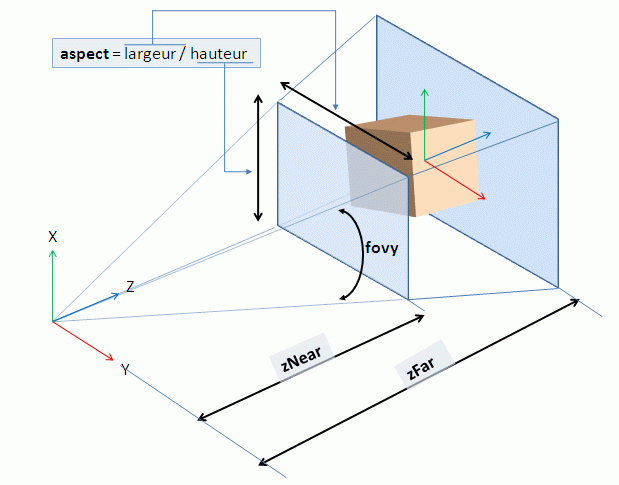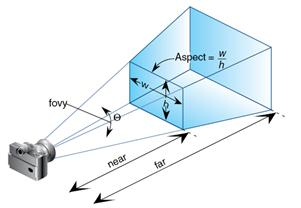### 正交投影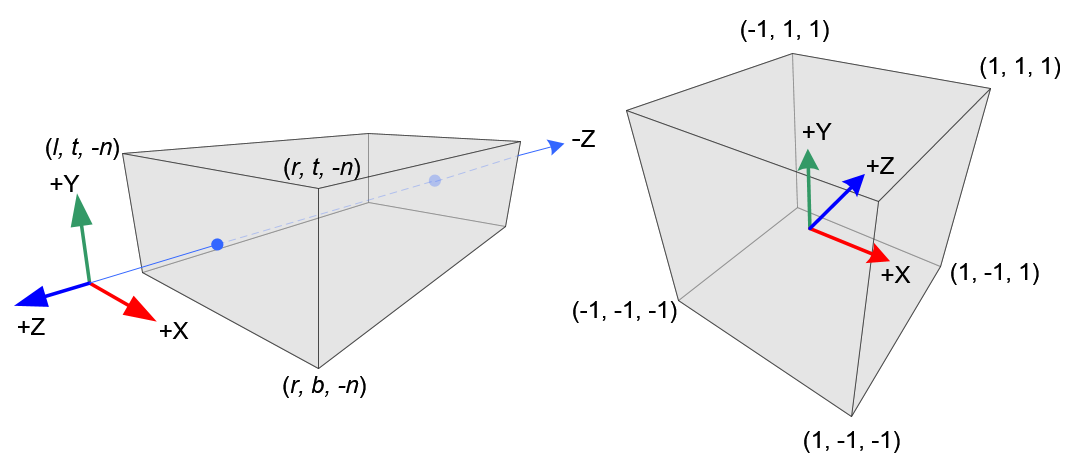$x_e$$x_n$的映射关系 [left , right] $\iff$ [-1 , 1]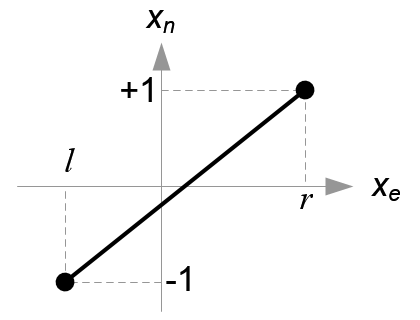$y_e$$y_n$的映射关系 [bottom , top] $\iff$ [-1 , 1]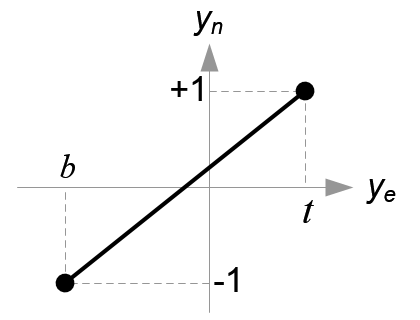$z_e$$z_n$的映射关系 [near , far] $\iff$ [-1 , 1]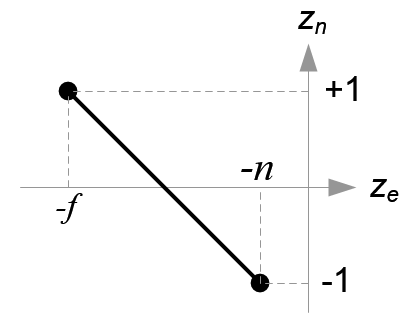## 视口变换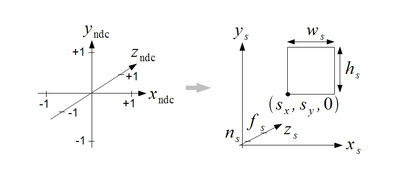//指定窗口的位置和宽高glViewport(GLint x , GLint y , GLsizei width , GLsizei height); //指定窗口的深度glDepthRangef(GLclampf near , GLclampf far);

$x_n$$x_s$的映射关系 [-1 , 1] $\iff$ [x , x + width]
$y_n$$y_s$的映射关系 [-1 , 1] $\iff$ [y , y + height]
$z_n$$z_s$的映射关系 [-1 , 1] $\iff$ [near , far]

X分量线性方程 $\frac {width} {2}$ x + $\frac {width} {2}$
Y分量线性方程 $\frac {height} {2}$ y + $\frac {height} {2}$
Z分量线性方程 $\frac {far - near} {2}$ $\frac {far + near} {2}$

## 参考文档• 私有
• 公开
• 删除